Select Page

# Optimization of Heater Zone Layout for a Rotating Susceptor in a Cold-wall MOCVD Reactor

case study

This case study describes the development of model-based temperature control of the susceptor of a Metal-Organic Chemical Vapor Deposition (MOCVD) reactor. A generic axisymmetric geometry has been used together with representative process conditions to highlight the issues related to the control of process temperature.

## Background

MOCVD is a deposition technique used to grow thin films on solid substrates (wafers) using organo-metallic compounds as sources. The films grown by MOCVD are usually semiconductors and are primarily used for the fabrication of electronic and optoelectronic devices that are components of mobile phones, optical communication, optical storage, light emitting diodes (LEDs), and solar cells. MOCVD is used to build up multiple layers of different materials, each of a precisely controlled thickness, to engineer a material with desired optical and electrical properties. One of the most important aspects in the MOCVD process for LED production is stringent temperature control. The color of the light emitted by an LED is a strong function of the substrate temperature during the deposition process. The wafer surface temperature uniformity directly translates into emission wavelength uniformity. To achieve a high yield, temperature control must be accurate, with very high wafer-to-wafer (WTW) and within wafer (WIW) temperature uniformity.

## How Many Independent Heaters Are Needed?

In this case study, we discuss a method for estimating the minimum number of independent heaters that are needed to attain the desired steady-state temperature uniformity over the susceptor radius for a range of process conditions. Since each independent heater requires a separate power supply, minimizing their number is important from the viewpoint of lowering hardware costs.

## Thermal Model of Reactor

Our Model-Based Control approach begins with developing physics-based models of the system to be controlled. Here, the system consists of a vertical, axisymmetric reactor with top-side showerhead and rotating susceptor heated from below by six heaters with uniform flux that may be operated independently or in combination as “heater zones”, as shown in the schematic within the dashed box in Figure 1. The heaters may be resistive films, lamp arrays, hot filaments, or RF inductive elements.Figure 1: Left: Schematic of 2D axisymmetric MOCVD reactor with susceptor heated by six heaters from below. Right: Process window.

From a control system viewpoint, the system input is composed of the set of heat fluxes, qi(r), corresponding to the ith heater zone, and the outputs are the temperatures T(r) measured at various radial points along the susceptor. The goal is to adjust the control flux, q(r), to obtain good temperature uniformity on the reaction surface.

The process gases enter through the showerhead at the top and exit through the annular exhaust at the outer boundary of the base of the reactor. In this thermal model, we have considered only the carrier gas, hydrogen, and not modeled the chemistry. The nominal operating process temperature is 1100°C, and the reactor pressure range is 100 Torr – 500 Torr with a flow rate in the range is 5 slm – 50 slm (slm = standard liters per minute). The process window defined by the ranges of flow rate and pressure is shown in the graph on the right of Figure 1. The susceptor rotation rate is assumed to be at 100 RPM. In this study, we specify the required temperature uniformity to be within ± 0.5°C over as much of the susceptor surface as possible. The heat transfer modes are:

• Gas Convection and Conduction
• The cold gas (27°C) is injected at the showerhead.
• Convective cooling flux of the susceptor is of order 10,000 W/m2.
• qc = h (Tsus-Tgas), h is of order 10-100 W/m2/K.
• qr = ε σ (Tsus4 – Twall4 ), ε = effective emissivity, σ = Stefan-Boltzmann constant.
• Radiation losses are of order 105 W/m2, but do not vary with flow rate or pressure.
• Conduction
• Gas conduction is fairly high for hydrogen compared to other gases.
• Solid conduction in susceptor is important, especially when heated from the backside.

A two-dimensional (2D) axisymmetric finite element model (FEM) of the reactor chamber was developed using COMSOL. Since the model contains swirl, it is actually a 3D flow, but is represented by a 2D mesh. The model incorporates conduction, convection and radiation heat transfer throughout the chamber. The flow model incorporates non-isothermal flow, with swirl and buoyancy effects. The model was run in open-loop for the baseline case of chamber pressure of 100 Torr and hydrogen flow of 50 slm. Identical control power was applied to each of the five inner zones, with the outer zone having five times this flux in order to compensate for losses which are higher at the circumference of the susceptor. The graphs on the left of Figure 2 show the radial temperature profile, along with normalized zone powers. The uniformity falls off rapidly after 0.13 m. Temperature gains were computed using this model.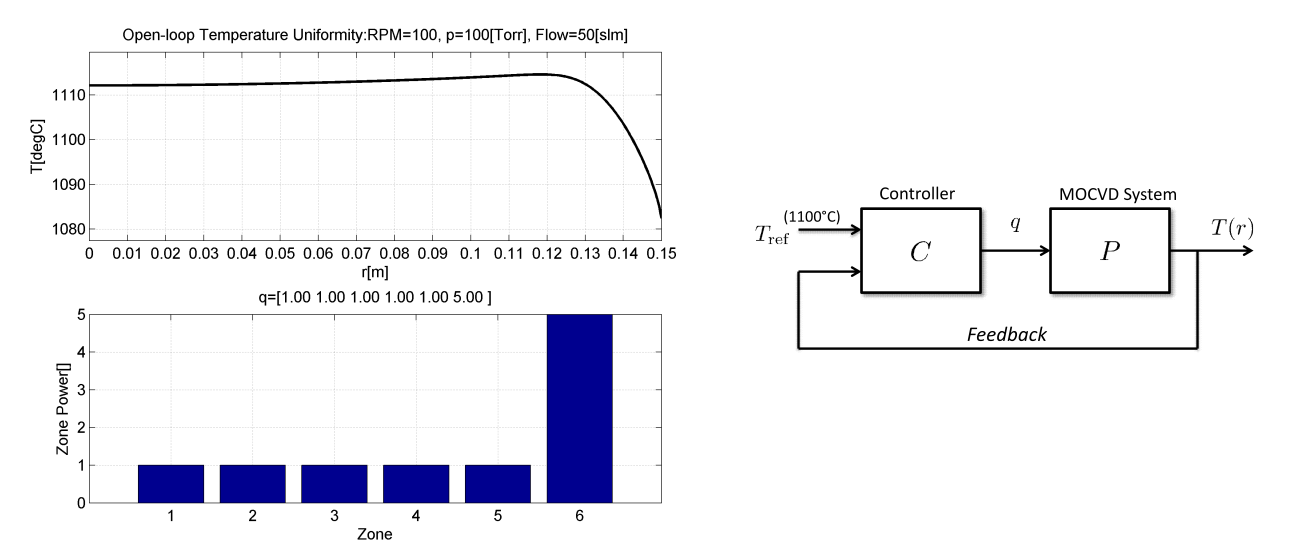Figure 2: Left: Baseline open-loop system performance. Right: Control architecture schematic.

## The Control Problem

The schematic on the right of Figure 2 shows the basic control architecture. The controller, C, adjusts the flux, q, to make the temperature T on the reaction surface uniform at the reference temperature, Tref . It is an “inverse problem” where we seek the inputs required to produce a given output. Real-time feedback is a common method of dynamically solving this problem. While we consider only the steady-state case here, the same methods are used to solve the time-varying dynamic control problem (e.g., uniformity during temperature ramp, stabilization, etc.).

The control problem may be framed as follows. Determine the input control flux, q, for each heater that produces the best temperature uniformity while:

• subject to the constraint that q ≥ 0,
• temperature uniformity is optimized over limited radius (r < Rmax).

In mathematical terms, the problem is stated as:## Maximum Feasible Area for Temperature Control

We know that there is a sharp drop-off at the edge of the susceptor due to increased heat losses. So we first solve this control problem with independent heaters in order to establish the limits of performance with regard to temperature uniformity, i.e., determine the maximum radial position on the susceptor (Rmax) up to which we can obtain ± 0.5°C uniformity at the nominal Tref  of 1100°C. The results are shown in the plots in Figure 3. It is clear that the design uniformity requirement can be met for Rmax = 0.13 m. Any attempt to optimize T over the entire susceptor (0 < r < 0.15 m) results in a significant area of the susceptor falling outside the design limits. For the remaining analysis we will only control the temperature for radius r < 0.13 m.Figure 3: Steady-state control for maximizing temperature uniformity with the six heaters for various values of maximum radius considered for control (pressure = 100 Torr, flow rate = 50 slm).

## Results with Single Zone Temperature Control

Having determined the “optimal” Rmax that produces the largest area of temperature within the design limits, we adopt a strategy whereby we fix the ratio of each heater power from the baseline optimal result (100 Torr, 50 slm), and scale this power distribution up and down using a single input scaling factor. This ratio approach may be implemented in hardware (e.g., filament density distribution, or resistance variation, etc.). Additionally, we want to optimize the input flux distribution for different operating conditions.

At first we consider a single zone control scheme that requires only one temperature sensor. We subsequently attempt improvements by increasing the number of independent control zones. Results with single-zone control are shown in Figure 4 for a process window shown earlier in Figure 1. In all cases the mean temperature optimized for r < 0.13 m and Tref = 1100°C. Except for the baseline case (100 T, 50 slm), the uniformity is poor. The reason for this poor temperature uniformity is the effect of convective heat transfer as described next.Figure 4: Results with one-zone control show poor uniformity over the process window.

To better understand the reason behind the temperature non-uniformity, we examine the flow patterns at various operating points that are shown in the contour plots of Figure 5. Figure 5 (a) shows the streamlines and temperature distribution for the baseline operating conditions. At a lower flow rate of 5 slm but at the same pressure of 100 Torr (Figure 5(b)), the change in the gas velocity distribution is small and so is its effect on convective heat transfer. However, at a pressure of 500 T (see Figure 5 (c) and Figure 5 (d)), buoyancy-driven flow creates recirculation cells that result in significant changes in the gas velocities (hence Nusselt number changes at susceptor surface), which in turn results in a redistribution of the convective losses. Hence single-zone control cannot work for this range of operating conditions.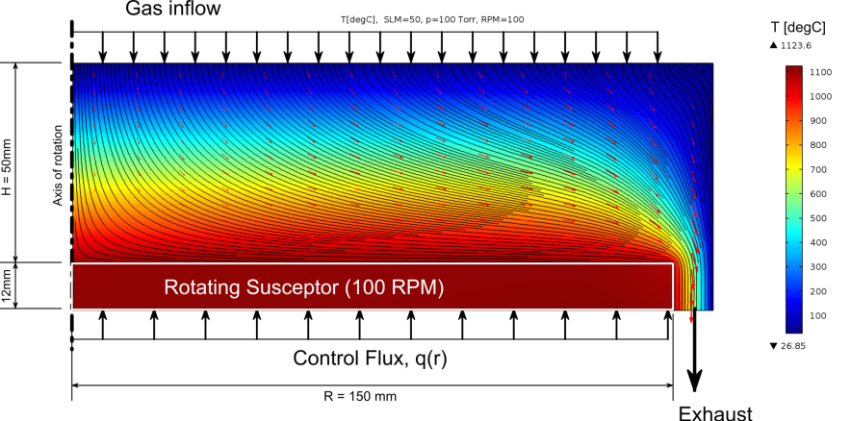(a)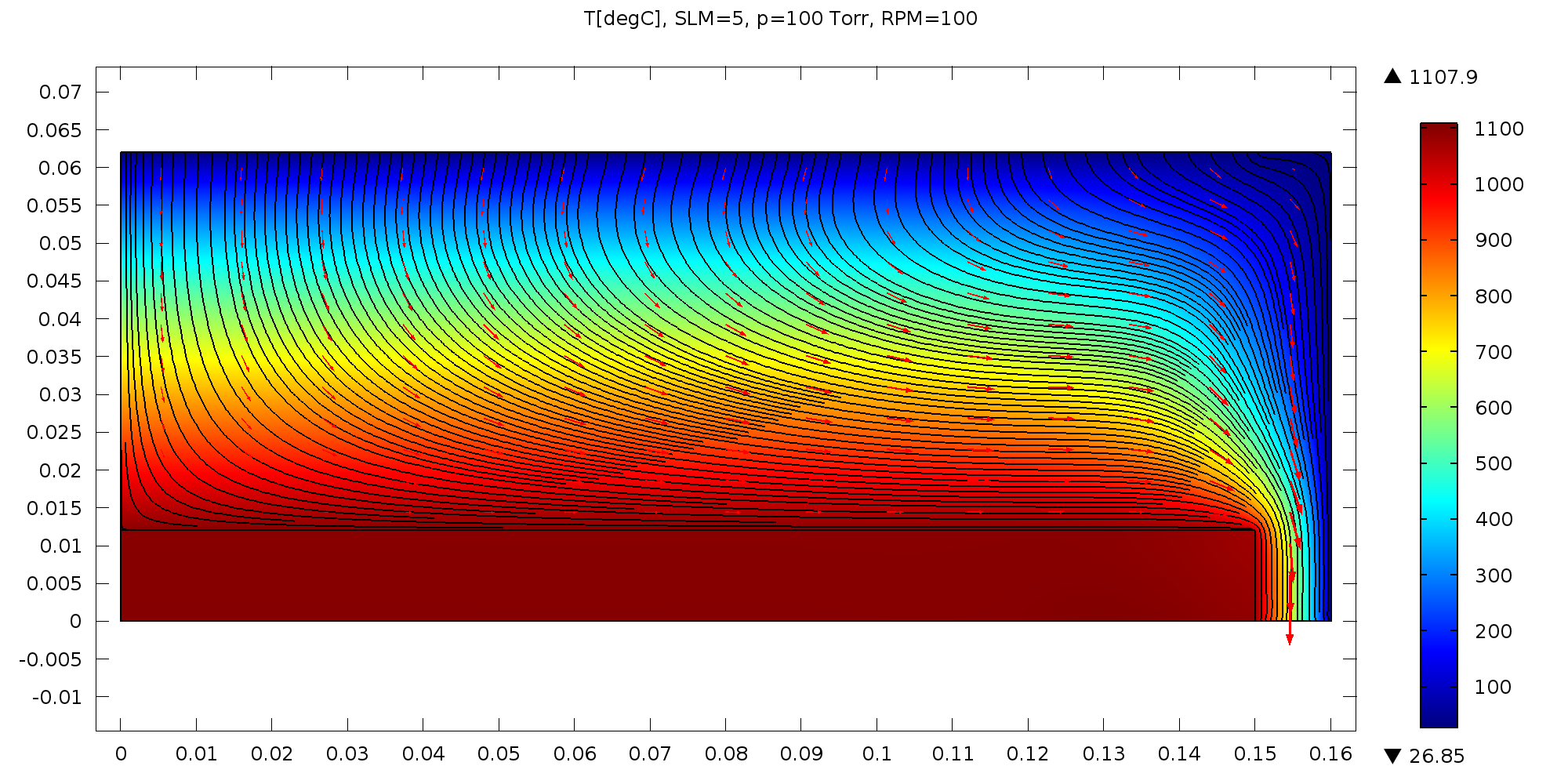(b)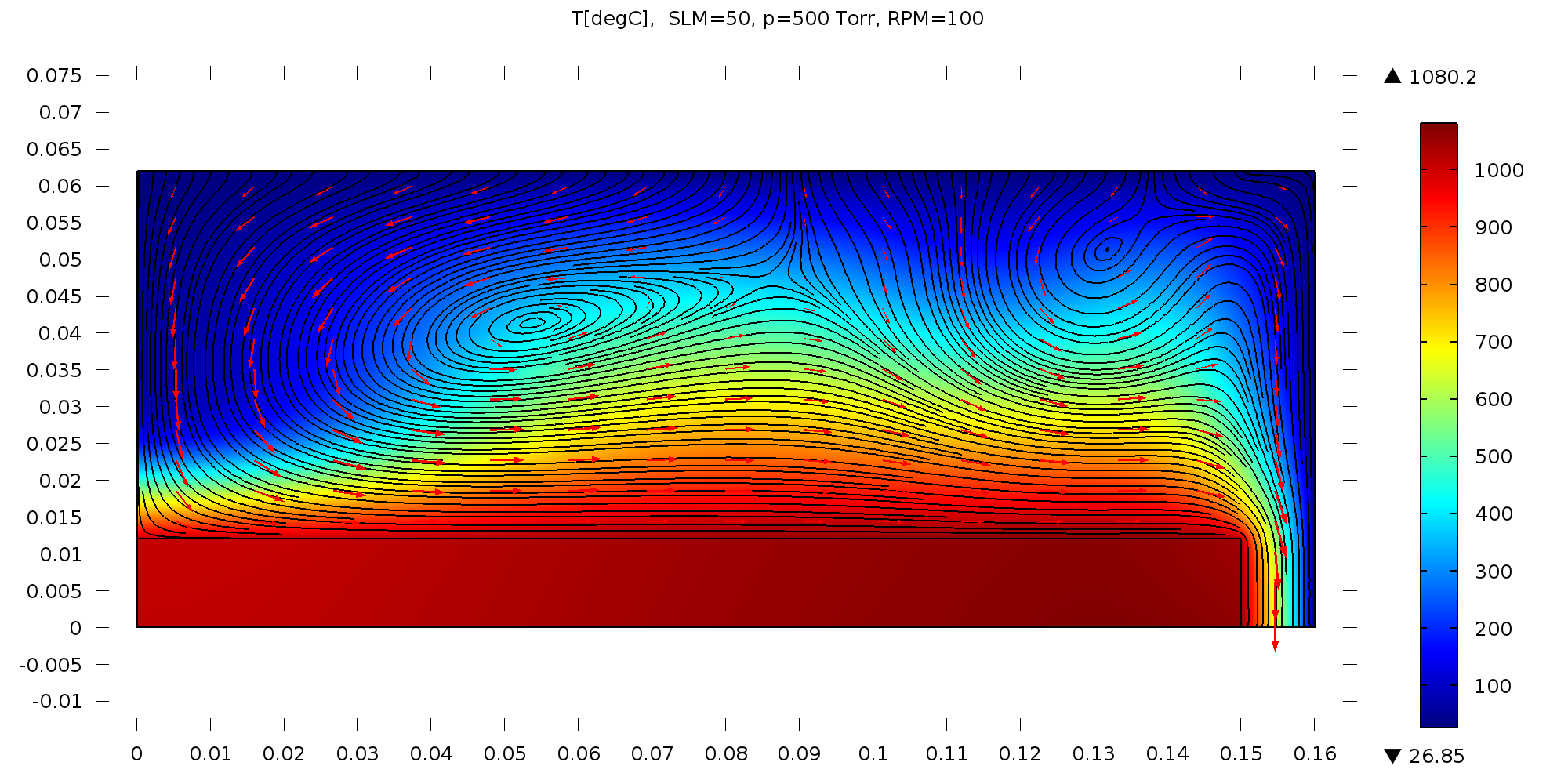(c)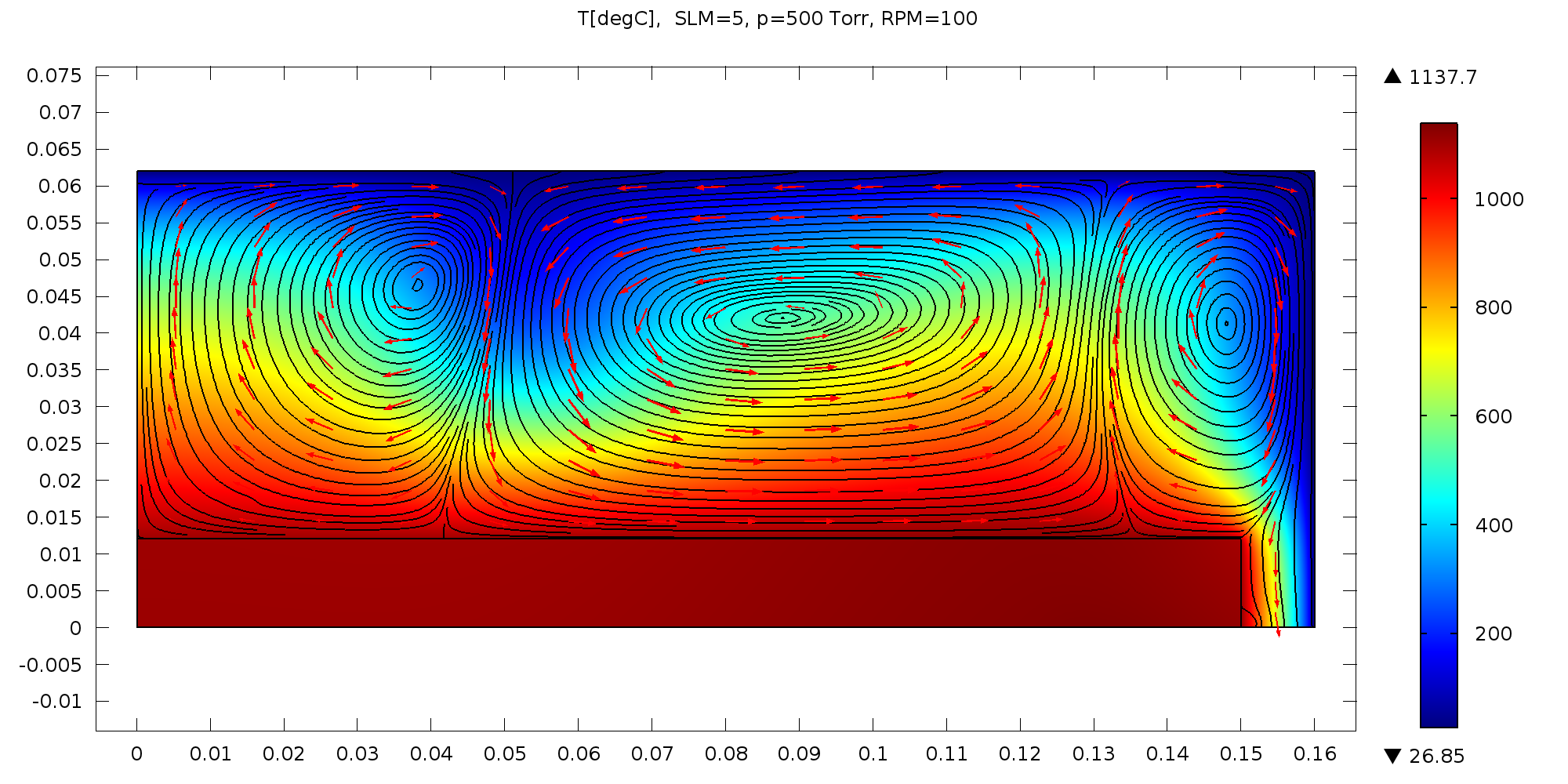(d)

Figure 5: Streamlines and temperature contours of flow in the MOCVD reactor modeled with COMSOL for various operating conditions in the process window. Recirculation cells form at higher pressures resulting in strong radial variation in convective heat transfer from the susceptor to the gas.

## Results with Two-Zone and Six-Zone Control

Since single zone control did not meet the design requirements, the next candidate was a two-zone scheme. Here, the inner five heaters are grouped together. The results are shown in Figure 6. The temperature uniformity shows some improvement, with both 100 Torr processes being close to being within the design limits. However, at higher pressures the uniformity is still not within design limits. Finally, a six-zone control scheme was adopted with each heater being controlled independently. The results shown in Figure 7 demonstrate that the design requirements are indeed satisfied with this configuration. The temperature uniformity is much better than the specification over most of the area. If the uniformity requirement is relaxed slightly, then fewer independent heater zones would be needed.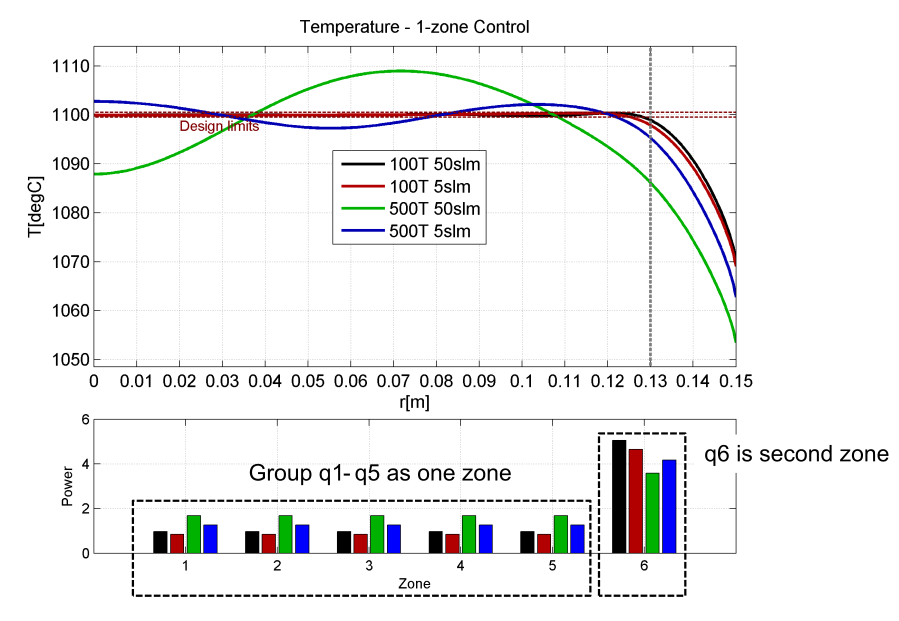Figure 6: Results with two-zone control. Better temperature uniformity is obtained compared to one-zone control but is still outside design specifications at high pressures.Figure 7: Results with six-zone control yield the best temperature uniformity over the process window.

## Benefits

In this study, we show how model-based control and optimization techniques may be used for optimal equipment design. We have successfully implemented such controllers for several commercial systems. If you would like more information regarding temperature control for your system, please contact us at (408) 617 4525.